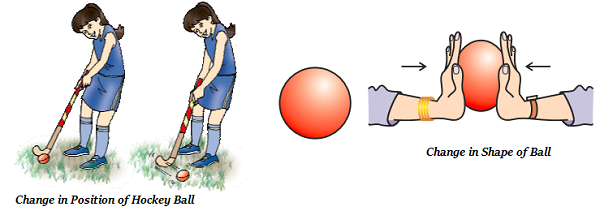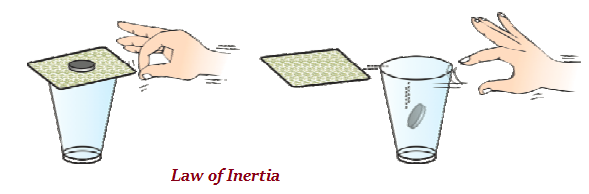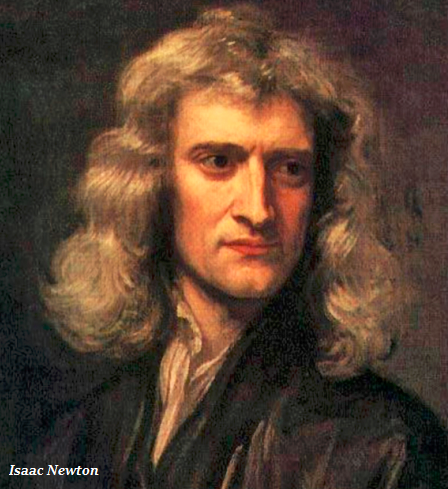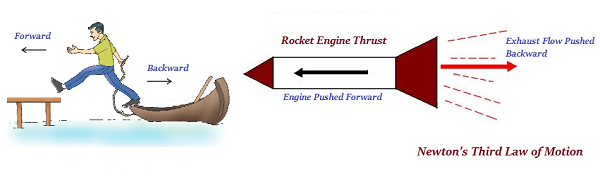# Physics - Force and Laws of Motion

## Introduction

• If we apply a force on an object, it may change its position or/and shape as well (as shown in the image given below).• Galileo Galilei and Isaac Newton explained a different approach to understand motion and applied force.

## First Law of Motion

• According to Galileo an object moves with a constant speed when no force acts on them.

• According to Newton’s First Law of Motion, “an object remains in a state of rest or of uniform motion in a straight line unless compelled to change that state by an applied force.”

• The tendency of uninterrupted objects to stay at rest or to keep moving (if in motion) with the same velocity is known as inertia.

• Newton’s first law of motion is also popular as the law of inertia.• As shown in the image given above, when the playing card is flicked by the finger, the coin placed on it falls in the glass; it explains the law of inertia.

• Therefore, inertia is a natural tendency of any object to resist a change in its state of motion or of rest.• Quantitatively, the inertia of an object is measured by its mass, as the heavier or bigger objects have greater inertia and lighter or smaller objects have lesser inertia.

## Second Law of Motion

• The second law of motion states that “the rate of change of momentum of an object is proportional to the applied unbalanced force in the direction of the force.”

• The momentum (represented as p) of an object is defined as the product of its mass (represented as m) and velocity (represented as v).

• Likewise, Momentum (m) = Mass (m) × Velocity (v).

• Momentum possesses both the direction as well as magnitude.

• The SI unit of momentum is represented as kilogram-meter per second (kg m s-1).

• The second law of motion illustrates a method to measure the force, which is acting on an object as a product of its mass and acceleration.

## Third Law of Motion

• The third law of motion states that – “to every action there is an equal and opposite reaction.”• It is important to remembered that the action and reaction always act on two different objects.

• It is important to remember that action and reaction forces are always equal in magnitude, but these forces may not produce accelerations of equal magnitudes because each force acts on a different object, which may have a different mass.

## Conservation of Momentum

• The conservation of momentum states that, in a given area, the amount of momentum remains constant.

• The momentum is neither created nor destroyed; however, it can be changed through the action of forces (described by Newton's laws of motion).

• The mass of an object multiplied by the velocity of the object is known as momentum.